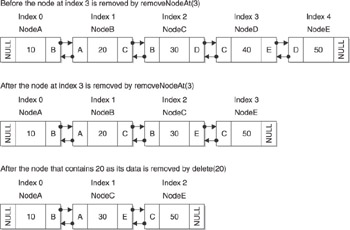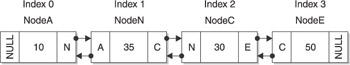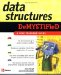# Enhanced LinkedList Class Using C

## Enhanced LinkedList Class Using C++

You ve seen how enhancements to the LinkedList class individually work; now we ll take a look at the entire application. I ve divided the following application into three files: the demo.cpp file, the LinkedList.h file, and the LinkedList . file. All three files are shown in the following code listing. You can use the LinkedList.h file and the LinkedList . cpp file, along with the specific files for queues and stacks that you learned about in Chapter 7 and Chapter 8.

The demo.cpp file contains the C++ application that uses the enhanced LinkedList class to create and manipulate a linked list. The LinkedList.h file contains definitions of the node and of the LinkedList class. The LinkedList .cpp file contains the definitions of member functions of the LinkedList class. All three files are shown next .

The demo.cpp file is where all the action takes place. As you ll see in the following example, the application begins by declaring an instance of the LinkedList class and then assigning the instance to a reference call list.

Next, the appendNode() function is called five times. The appendNode() function is an original member function of the LinkedList class and appends a new node to the linked list. The linked list shown at the top of Figure 9-4 is the linked list after the last appendNode() function is called.Figure 9-4: The top is the linked list before the node is removed, the middle is after removeNodeAt(3) is called, and the bottom is after deleteNode(2) is called.

Once the linked list is created, the application calls the removeNodeAt(3) function to remove the node located at index 3. The middle linked list in Figure 9-4 shows the status of the linked list after the removeNodeAt(3) function executes.

The application then calls the findNode(20) function to locate the index of the node that contains 20 as its data element. Based on the linked list shown at the bottom of Figure 9-4, the findNode(20) function returns the index value 1.

The deleteNode(20) function is then called and removes the node from the linked list that has 20 as the value of its data element. The linked list shown at the bottom of Figure 9-4 illustrates the linked list after the deleteNode(20) function is called.

A new node is then inserted into the linked list by calling the insertNodeAt(1, 35) function. This function inserts a new node at index 1 in the linked list and assigns 35 to the data element of the node. Figure 9-5 is the linked list after the insertNodeAt(1, 35) function is called.

The peek(3) function is called to retrieve the value of the node in the third index position of the linked list. Based on the linked list shown in Figure 9-5, the peek(3) function returns 50 as the data value of the node in index position 3.Figure 9-5: The linked list after the insertNodeAt(1) is called

The last function called is getSize() , which returns the size of the linked list. As seen in Figure 9-5, the linked list has four nodes; therefore, the getSize() function returns the value 4.

The last statement in the demo application uses the delete operator to remove the instance of the LinkedList class from memory.

` //demo.cpp #include <iostream> using namespace std; void main(){  LinkedList* list = new LinkedList();  list->appendNode(10);  list->appendNode(20);  list->appendNode(30);  list->appendNode(40);  list->appendNode(50);  list->removeNodeAt(3);  int index = list->findNode(20);  list->deleteNode(20);  list->insertNodeAt(1, 35);  int data = list->peek(3);  int size = list->getSize();  delete list; } //LinkedList.h typedef struct Node{  struct Node(int data)  {  this->data = data;  previous = NULL;  next = NULL;  }  int data;  struct Node* previous;  struct Node* next; } NODE; class LinkedList {  protected:  NODE* front;  NODE* back;  int size;  void removeNode(NODE* node);  public:  LinkedList();  virtual ~LinkedList();  void appendNode(int);  void displayNodes();  void displayNodesReverse();  void destroyList();  void removeNodeAt(int);  int findNode(int);  void deleteNode(int);  void insertNodeAt(int,int);  int peek(int);  int getSize(); }; //LinkedList.cpp #include "LinkedList.h" CLinkedList::CLinkedList() {  front = NULL;  back = NULL;  size = 0; } CLinkedList::~CLinkedList() {  destroyList(); } void CLinkedList::appendNode(int data) {  NODE* n = new NODE(data);  if(back == NULL)  {  back = n;  front = n;  }  else  {  back->next = n;  n->previous = back;  back = n;  }  size++; } void CLinkedList::displayNodes() {  cout << "Elements: ";  NODE* temp = front;  while(temp != NULL)  {  cout << temp->data << " ";  temp = temp->next;  }  cout << endl; } void CLinkedList::displayNodesReverse() {  cout << "Elements: ";  NODE* temp = back;  while(temp != NULL)  {  cout << temp->data << " ";  temp = temp->previous;  }  cout << endl; } void CLinkedList::destroyList() {  NODE* temp = back;  while(temp != NULL)  {  NODE* temp2 = temp;  temp = temp->previous;  delete temp2;  }  back = NULL;  front = NULL; } void CLinkedList::removeNode(NODE* node) {  if(node->previous == NULL && node->next == NULL)  {  back = NULL;  front = NULL;  }  else if(node->previous == NULL)  {  front = node->next;  node->next->previous = NULL;  }  else if(node->next == NULL)  {  back = node->previous;  node->previous->next = NULL;  }  else  {  node->previous->next = node->next;  node->next->previous = node->previous;  }  delete node;  size--; } void CLinkedList::removeNodeAt(int index) {  if(index < 0  index > size-1)  {  return;  }  NODE* temp_node = front;  for(int i=0; i<index; i++)  {  temp_node = temp_node->next;  }  removeNode(temp_node); } int CLinkedList::findNode(int data) {  int index = 0;  NODE* temp_node = front;  while(temp_node != NULL)  {  if(temp_node->data == data)  {  // return the index of the node  return index;  }  else  {  temp_node = temp_node->next;  index++;  }  }  return -1; } void CLinkedList::deleteNode(int data) {  NODE* temp_node = front;  while(temp_node != NULL)  {  if(temp_node->data == data)  {  removeNode(temp_node);  return;  }  else  {  temp_node = temp_node->next;  }  } } void CLinkedList::insertNodeAt(int index, int data) {  if(index < 0  index > size)  {  return;  }  NODE* new_node = new NODE(data);  if(size == 0)  {  front = new_node;  back = new_node;  }  else if(index == 0)  {  front->previous = new_node;  new_node->next = front;  front = new_node;  }  else if(index == size)  {  back->next = new_node;  new_node->previous = back;  back = new_node;  }  else  {  NODE* temp = front;    for(int i=0; i<index; i++)  {  temp = temp->next;  }  new_node->next = temp;  new_node->previous = temp->previous;  temp->previous->next = new_node;  temp->previous = new_node;  }  size++; } int CLinkedList::peek(int index) {   if(index < 0  index > size-1)  {  return 0;  }  NODE* temp = front;    for(int i=0; i<index; i++)  {  temp = temp->next;  }  return temp->data; } int CLinkedList::getSize() {  return size; } `Data Structures Demystified (Demystified)
ISBN: 0072253592
EAN: 2147483647
Year: 2006
Pages: 90

Similar book on Amazon